Version 12/2015 of MESYS shaft and rolling bearing calculation software is available for download now and offers several new possibilities. The software is available in six languages and is used in 24 countries worldwide.

## Calculation of harmonic response in shaft calculation

One often requested extension was the calculation of harmonic response.

Imbalance of a shaft will result in vibrations and dynamic bearing forces. Sometimes balance grades are given for an application like defined by ISO 1940. A balance grade G 6.3 according ISO 1940 would mean that eperm*ω = 6.3mm/s, so for larger rotation speed a smaller eccentricity is permitted. The dynamic forces are then F = mass*e*ω2. The problem is that the location of the force and the phase between multiple forces is not known, so often one force at the center of mass is considered.

There are applications where shafts and rotors are balanced and dynamic forces will be small compared to static forces; there are also applications where dynamic forces are much larger than the static forces. In the first case the contact point in the bearings will be stationary and in the second case the contact point in the bearings will rotate with the shaft.

Typically the equations of motion are solved in a fixed non-rotating frame Mx’’ + (G+C)x’ + Kx = F where M is the mass matrix, G is the speed dependent gyroscopic matrix, C is the damping matrix, K is the stiffness matrix and F is the load.

The MESYS shaft calculation like many other programs solves the static problem considering a nonlinear stiffness and then solves the linear modal problem using the tangent stiffness matrix at operating conditions: (Mλ2 + (G+C) λ+Kt)y = 0. If this equation is used to calculate harmonic response (as done by the software) it is only valid for small amplitudes or in case of a linear stiffness matrix.

If rolling bearings with clearance are used, the dynamic forces have to be smaller than the static forces. Dynamic forces larger than static forces would lead to a rotating contact point in the bearing and therefore the linear calculation with an anisotropic bearing stiffness matrix is not valid. In this case either a time integration would be required or a calculation in a rotating frame, both is not supported by the shaft calculation software. In a rotating frame imbalance would be a static load and the weight would be a dynamic load. General FEA programs or specialized rotor dynamic programs have to be used in this case.

If bearings with pretension are used, the stiffness matrix is almost isotropic and then dynamic forces larger than static radial forces would be possible, still the nonlinear behavior of the bearing is ignored.

As an example a simple rotor is used. An imbalance of 5gmm is considered on the left side for a rotation speed of 20000rpm. This would lead to a dynamic force of . The calculated harmonic response at 20000rpm is 30.9N for the left bearing and just 6N for the right bearing. Note that the difference is larger than the calculated value of the dynamic force of 21.93N, this is because of the dynamic forces of the shaft.The harmonic response is speed dependent. The bearing forces will rise if the rotation speed approaches the natural frequency.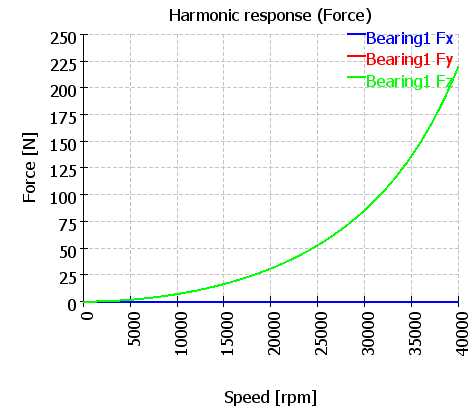A diagram showing bearing forces over time for the given speed is available too, there the opposite direction of both forces can be seen: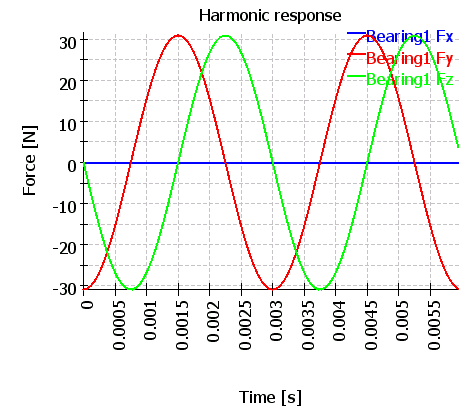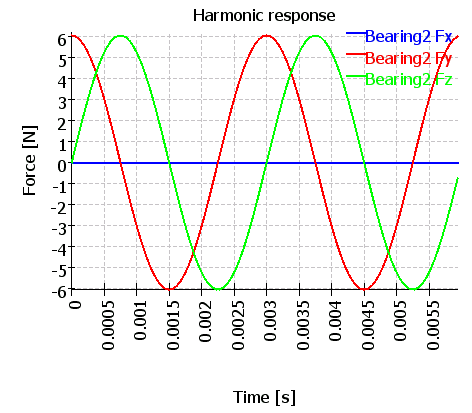As the bearings have an isotropic stiffness matrix, the amplitudes in y/z-directions are the same. If the amplitudes would be different and larger than the static forces, the assumptions of the calculation would not be valid.

In the current version the harmonic response is not considered in the calculation of bearing life, so it only provides the force amplitudes to be expected.

## Improved parameter variations in shaft and bearing analysis

Parameter variations are a helpful tool to visualize the influence of certain parameters on results.

As input for the calculation, additional rules can be specified. The simple rotor from above is used again and the axial clearance for bearing 2 is set to the same value as the axial clearance of bearing 1.

Axial clearance of the bearings and the shaft speed are varied in a given range: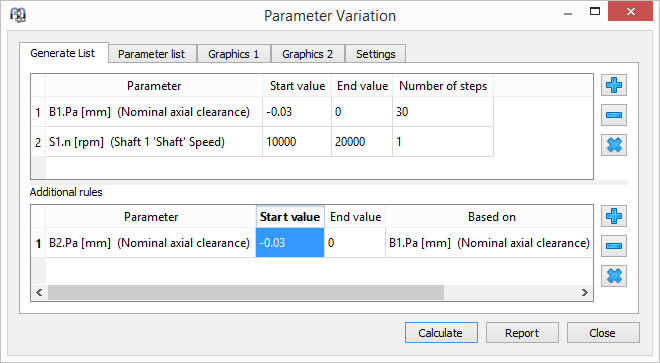In the result graphics a second axis was added. So several results can be shown in the same diagram like the minimal bearing life and spin-to-roll ratio in the following image. Bearing life and spin-to-roll ratio are shown for two rotation speeds of the shaft.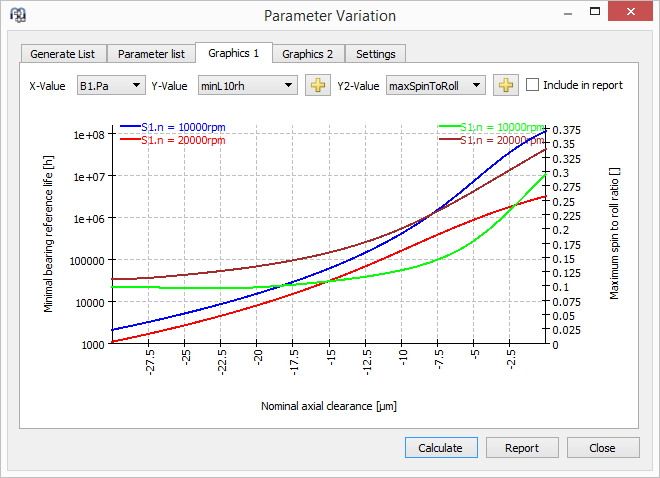## Gyroscopic moment for high speed ball bearings

Finally the gyroscopic moment on ball bearings is considered in addition to the centrifugal forces on the balls. The gyroscopic moment is taken by friction forces on inner and outer race contacts. In case of different contact angles on inner and outer race, these friction forces affect the normal forces and therefore the bearing life.

The software offers several options for the distribution of these forces. For example for outer race control either only a frictional force on the outer race can be considered or a force on both contacts proportional to the normal forces. Currently five different options are available.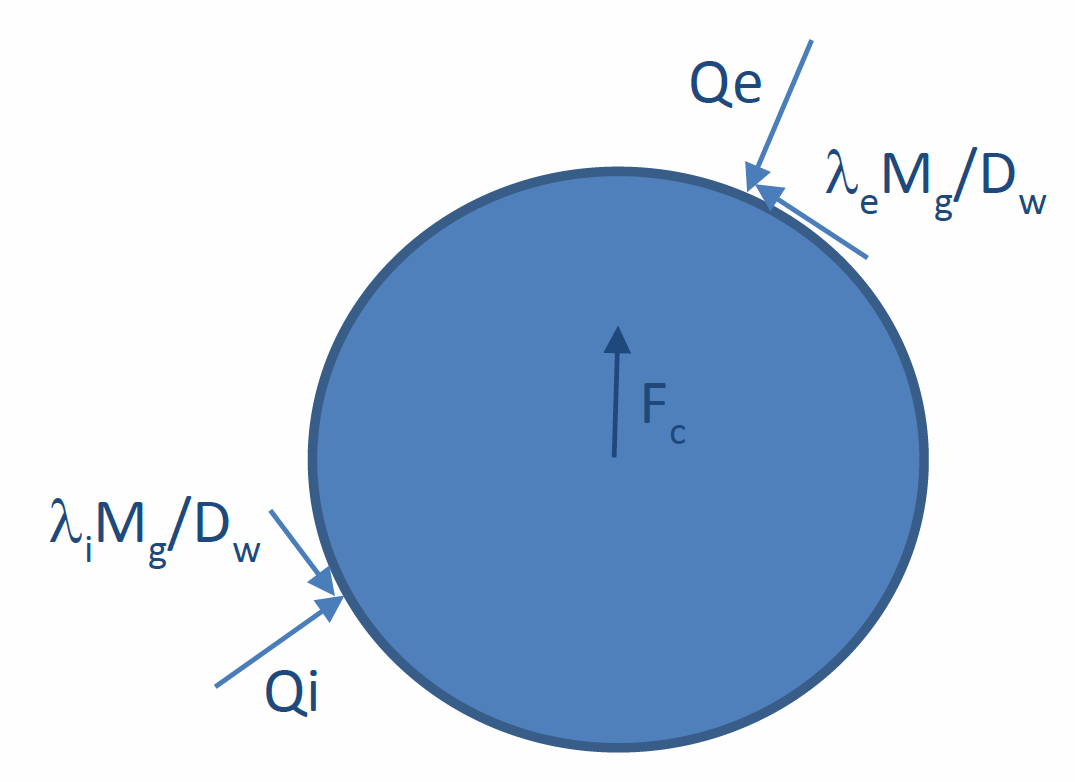The influence of the gyroscopic moment acting on the balls increases with contact angle and orbit speed. It only affects normal forces if the contact angle on inner and outer race contact are different.

## Centrifugal force on radial cylindrical roller bearings

Centrifugal forces can now be considered for radial cylindrical roller bearings too. Note that centrifugal forces are activated as default. It will be disabled if old files are loaded, so the results are not changed. Calculation time will be increased if centrifugal forces are considered.

## Calculation of life for rolling element set

Usually fatigue in rolling bearings will be seen on the races first. In case of high strength materials for bearing races combined with standard rolling elements, the rolling elements could fail first. To take this into account the calculation of rolling element life analogous to ISO/TS 16281 was added in the bearing calculation. The local load capacity Qc is calculated as for the bearing races using changes because of loaded volume and load cycles. Because of higher hardness the rolling elements should have a higher load capacity as the races, this could be considered by material factors. For ball bearing the calculation assumes contact on one circle only, so real life should be larger than calculated.

This calculation is disabled by default and can be enabled with a selection of Weibull exponents to be used.

## Calculation of lubricant temperature

The lubricant temperature can optionally calculated as mean value of inner and outer ring temperature defined on page loading.

## Calculation of pressure viscosity coefficient

The pressure viscosity coefficient can now be calculated according ISO 1281 or AGMA 925 if the lubrication is set to user input.

This allows to take the temperature dependency into account in case of load spectra calculations with varying temperature.

The pressure viscosity coefficient is only used to calculate lubricant film thickness.